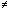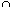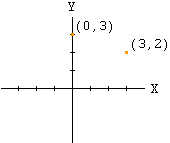Date: Thu, 2 Sep 1999 20:33:21 EDT My name is Judy. I guess the level on this would be about middle school, and I am an other!! I've been out of school for alot of years. I have a couple of questions, I guess. What does an equal (=) with a slash through it mean? I put intersects, but I think I'm wrong. What is the symbol of a small n? Only the symbol is wider. Example: If A = {1.2} B = {2,3} then what is A (n) B? Can you make sense out of that? What is the equation of the y-axis? I can give you more! It is algebra ( I think) with all the x and y to the second and third power. I am 40 years old, and I work for the USDA. I signed up with the distance learning to earn 40 credits. I had to take this math test to try to earn a prerequisite. If I pass, I can take the good classes. If not, I will have to struggle through the icky math course!!! If you could give me some examples of the x and y stuff, it would help a lot. I only have 60 questions of several kinds of math. I have already answered 42. So, as you can see, I'm not looking for a free ride. Please help Judy Hi Judy Math courses aren't icky. They are fun and exciting and open doors to all sorts of other subjects. The equal sign with a slash through it,means not equal. The symboldesignates intersection. It is a symbol used between two sets and it designates the elements that are common to the two sets. Thus, for example, if P = {1,3,5,6} and Q = {2,3,4,5} then PQ = {3,5} In the Cartesian coordinate system the points (x,y) on the y-axis have the x-value equal to zero, ie x = 0. The equation of the y-axis is x = 0.Cheers Penny

Go to Math Central